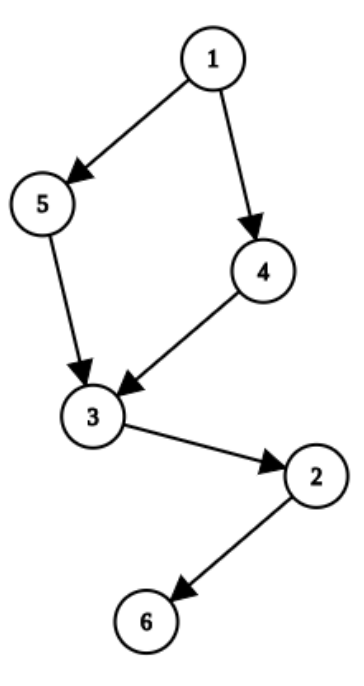시간 제한메모리 제한제출정답맞힌 사람정답 비율
1 초 512 MB0000.000%

## 문제

Let G be a directed acyclic graph. If c1, c2, c3, . . . cn are distinct vertices of G such that there is a path from c1 to c2, there is a path from c2 to c3, . . . and there is a path from cn−1 to cn, we say that array C = (c1, c2, c3, . . . cn) is an ordered array starting at c1 and ending at cn. Note that between neighbouring elements ci and ci+1 of ordered array C it isn’t necessary to exist a direct edge, it is enough for the path to exist from ci to ci+1.

For this definition of an ordered array C = (c1, c2, c3, . . . cn), we define its length len(C) = n. Therefore, the length of an ordered array is equal to the number of vertices it holds. Note that the ordered array can have a length of 1 when holding a single vertex which represents both its beginning and its end.

Also, for an ordered array C = (c1, c2, c3, . . . cn) we can define its sign as sgn(C) = (−1)len(C)+1. For vertices x and y of G, let’s denote with Sx,y a set of all ordered arrays that start in x and end in y.

Finally, we define the tension between nodes x and y as tns(x, y) = ΣC∈Sx,ysgn(C). Therefore, the tension between nodes x and y equals the sum of signs of all ordered arrays that start in x and end in y.

An integer K is given. Your task is to construct a directed acyclic graph with at most 1000 vertices and at most 1000 edges for which tns(1, N) = K holds. Number N in the previous expression denotes the number of vertices in a graph. Vertices of a graph should be indexed using positive integers from 1 to N.

## 입력

The first line contains an integer K (|K| ≤ 1018) from the task description.

## 출력

In the first line you should output the number of vertices and the number of edges of the constructed graph. Let’s denote the number of vertices of that graph with N (1 ≤ N ≤ 1000), and the number of edges with M (0 ≤ M ≤ 1000).

In the i-th of the next M lines you should output two distinct integers Xi and Yi (1 ≤ Xi, Yi ≤ N), which represent the i-th edge which is directed from vertex with index Xi towards vertex with index Yi. Each edge must appear only once in the output.

Also, the absolute value of tension between each two nodes in the graph must be less or equal to 280.

If there are multiple solutions, output any of them.

## 예제 입력 1

0


## 예제 출력 1

6 6
1 4
1 5
4 3
5 3
3 2
2 6


The constructed graph has 6 vertices. Ordered arrays that start in 1 and end in 6 are: (1, 6), (1, 4, 6), (1, 5, 6), (1, 3, 6), (1, 2, 6), (1, 4, 3, 6), (1, 4, 2, 6), (1, 5, 3, 6), (1, 5, 2, 6), (1, 3, 2, 6), (1, 4, 3, 2, 6), (1, 5, 3, 2, 6). Their lengths are (in order): 1, 2, 2, 2, 2, 3, 3, 3, 3, 3, 4, 4, so their signs are −1, 1, 1, 1, 1, −1, −1, −1, −1, −1, 1, 1. Therefore, the tension between 1 and 6 is equal to −1 + 1 + 1 + 1 + 1 − 1 − 1 − 1 − 1 − 1 + 1 + 1 = 0.## 예제 입력 2

1


## 예제 출력 2

1 0


## 예제 입력 3

2


## 예제 출력 3

6 8
1 2
1 3
1 4
1 5
5 4
2 6
3 6
4 6